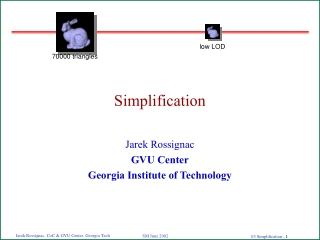DownloadDownload PresentationSimplification

# Simplification

Télécharger la présentation## Simplification

- - - - - - - - - - - - - - - - - - - - - - - - - - - E N D - - - - - - - - - - - - - - - - - - - - - - - - - - -
##### Presentation Transcript

1. Simplification low LOD 70000 triangles Jarek Rossignac GVU Center Georgia Institute of Technology

2. Quantize vertices Compress Decompress Binary format Loss-less or lossy compression? • Loss-less compression • Quantize parameters (coordinates) based on application needs • Finite precision measurements, design, computation • Limited needs for accuracy in some applications • Encode quantized location and exact incidence • Lossy compression • Encode approximations of the surface using a different representation • How to measure the error to ensure that tolerance is not exceeded? Lossy Loss-less

3. Triangle count reduction techniques (LOD) • Quantize & cluster vertex data (Rossignac&Borrel’92) • remove degenerate triangles (that have coincident vertices) • Adapted by P. Lindstrom for out-of-core simplification • Repeatedly collapse best edge (Ronfard&Rossignac96) • while minimizing maximum error bound • Adapted by M. Garland for least square error

4. Vertex clustering (Rossignac-Borrel) • Subdivide box around object into grid of cells • Coalesce vertices in each cell into one “attractor” • Remove degenerate triangles • More than one vertex in a cell • Not needed for dangling edge or vertex

5. Rossignac&Borrel 93

6. Rossignac&Borrel 93

7. Improving on Vertex Clustering • Advantages • Trivial to implement • Fast • Works on any mesh or triangle soup • Guaranteed Hausdroff error to diagonal of cell • Reduces topology • Removes holes. Never creates one • Merges connected shells components. Never splits them. • Drawbacks • Produces sub-optimal results • Too much error for a given triangle count reduction • Prevents the merging of distant vertices on flat portions of the surface • Fix: limit vertex moves by the resulting error • Not a fixed grid

8. Simplification through edge collapse

9. How to decide which edges to collapse? • Minimize the error between original and resulting LOD • How to compute/estimate error • Peformance • Geometric proximity clustering of vertices (pessimistic) • Rossignac&Borrel: quantizing vertices identifies candidate edges • Error is bounded by the quantization error • Fast, easy, robust, but sub-optimal results • Collapse edges • Longer edges in almost planar regions • Estimate error as max distance to supporting planes (Ronfard&Rossignac) • Must keep list of all planes supporting triangles incident on contracted edges • Use sum of squares instead of max (Heckbert&Garland): faster, no bound • L2 norm, needs only add 4x4 matrices when clusters are merged

10. N P Q P Q Distance and quadratic error • Point-plane distance • Point P=(x,y,z) • Plane containing point Qm and having unit normal Nm • Distance ||PQmNm|| • Can compute max (conservative, Ronfard&Rossignac) or sum (cheap, Heckbert&Garland) of (PQmNm)2 for the planes of all the triangles Tm incident upon vertices merged at P • Distance squared: (PQmNm)2 = amx2+bmy2+cmz2+dmxy+emyz+fmzx+gmx+hmy+imz+jm • Sum of distances squared: (PQmNm)2 + (PQnNn)2 = (am+an)x2 +(bm+bn)y2 +(cm+cn)z2 +(dm+dn)x +(em+en)y +(fm+fn)z +gm +gn • As vertices are merged recursively: • With max, you need to remember all the planes • With sum, you just add the coefficients

11. Ronfard&Rossignac EG’96

12. ET T Shape complexity • Optimal bit allocation in 3D compression • King&Rossignac, Computational Geometry, Theory & Applications’99 • Approximate ET by K/T • Assumes uniform error distribution (all edge collapses increase ET) • Assumes smooth shapes with no features smaller than tesselation • Use integral of curvature to estimate K • K estimate computed efficiently using sphere-fit for each edge • Formula derived for objects made of relatively large spherical caps • Yields crude estimate for doubly curved surfaces (saddle points...) K/T